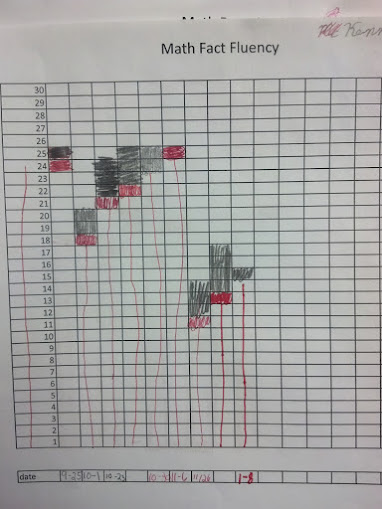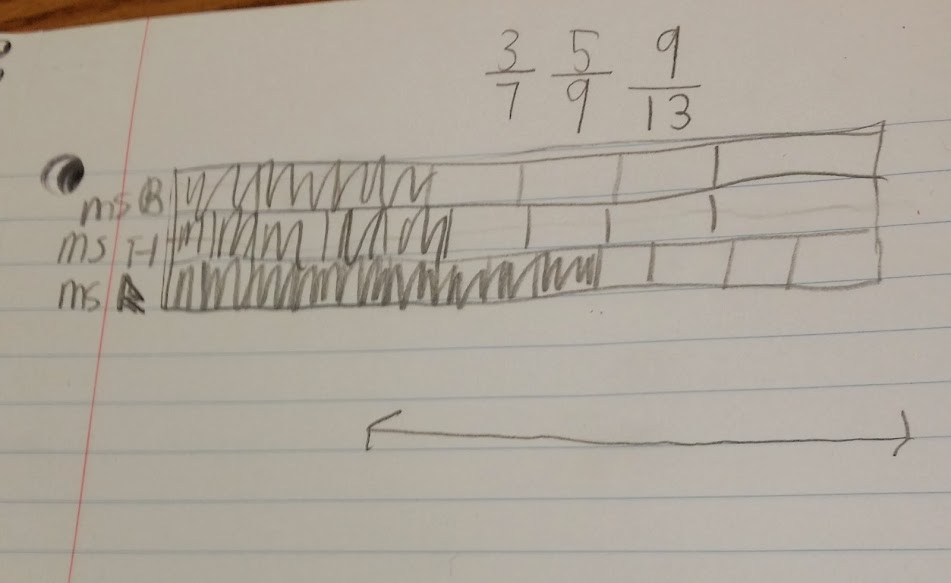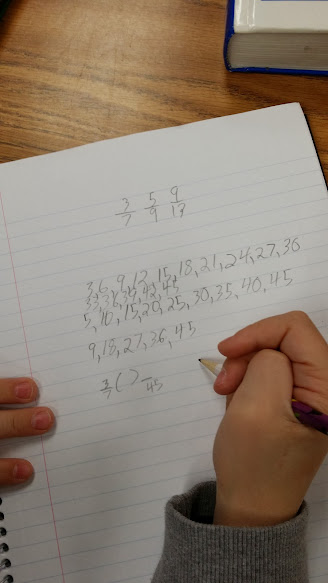# Recycling with Common Numerators

2 teachers like this lesson
Print Lesson

## Objective

SWBAT compare fractions by creating common numerators.

#### Big Idea

In this lesson students compare fractions by creating common numerators in an engaging problem about recycling.

## Warm UP

7 minutes

Students will start today's lesson with a fluency assessment.  This assessment is from Monitoring Basic Skills Progress Second Edition: Basic Math Computation by Lynn S. Fuchs, Carol L. Hamlett, and Douglas Fuchs.

This is an assessment I have my students do each week and then graph their results.  It allows them to reflect on their learning of basic math facts, as well as using all four operations with whole numbers, and adding and subtracting unit fractions.  (It also happens to be the quietest time in my math classroom all week!!)

This is what my classroom looks like as students work on this assessment.

This is sample of a student's graph. You can also click here to hear my thoughts about this graph below.Click here to see an example of a typical fourth grade fluency decrease since students are completing the fourth grade fluency set that incorporates division, multiplication, addition, subtraction, and adding and subtracting fractions with like denominators. (At this point, students have not had many fraction lessons, thus very very students are able to complete the fraction problems)

I do not start my students with the fourth grade skills, but at this point in the year, all students are using the fourth grade set.. I chose to start them with the end of the third grade skills which covers addition, subtraction and multiplication and division of basic facts. I strongly believe in a balanced math approach, which is one reason why I also believe in common core standards.  By having a balance of building conceptual understanding, application of problems, and computational fluency, students can experience rigorous mathematics.  I want to make clear that this assessment ONLY measures basic math computation.  It is only one piece of students' knowledge. The assessments in this book, for each grade level, do not change in difficulty over the course of the year.  Therefore, a student's increase in score over the school year truly reflects improvement in the student's ability to work the math problems at that grade level.

## Concept Development

45 minutes

I begin this lesson by reviewing the two fraction rules that the class came up with in previous lessons.  These are important fraction concepts that I want all students to know and be able to understand and use.

Rule #1 - If the numerators of the two fractions that we are comparing are the same, the fraction with the smaller number in the denominator always represents the bigger (greater) fraction

Rule #2 - If the denominators of the two fractions that we are comparing are the same, the fraction with the larger number in the numerator always represents the bigger (greater) fraction

I review how to find common denominators using the algorithm by asking students to use their whiteboards to compare 4/7 and 2/5.  I ask student partners to share with each other how they solved the problem.

Then I ask students to compare the fractions 6/8 and 5/7 by creating common denominators.  After doing so, I stop students and pose this questions:

If we can create common denominators to compare fractions by making equivalent fractions, can we also create common numerators?

I listen to students thoughts about this question.  Some students are quick to say yes, that can be done, while others say no.  I ask a student that responded yes to see if he/she could explain or give an example.

Then, I do several problems together with students on the board.  In this lesson I make sure to repeat several times that finding a common numerator can be a very useful strategy when comparing fractions, but when adding and subtracting fractions, finding the common denominator is the strategy that works. I list some examples on the board like 3/11 and 6/15.  I ask students to think about if we were comparing these fractions, why finding a common numerator might be more efficient.  Students quickly realize that they only need to multiply 3/11 by 2/2 to find a common numerators where as they will need to do more complex calculations to find a common denominator.

Next,  I teach how to play the game Two Truths & A Lie.  This game started out as an ice-breaker or a party game but I think it is fun to play in math, especially when trying to get students to make conjectures or prove their answers. The rules are simple. I list three comparison fraction numbers sentences on the board.  Two are true, and one is a lie or incorrect. The students must pick out the false number sentence.  I list:

4/5 < 8/9

4/6 < 3/5

7/8 > 6/7

Students share their thinking at the table groups. As students share, I circulate the room to observe students using this new strategy of finding common numerators and ask guiding questions.

For the remainder of the class students work on the Recycling Problem- Common Numerator  I allow students to work with their learning partner to solve the problem.  I instruct students that for this problem they must, with their partner, show three different ways, or three different strategies to solve this problem.  In this video, you can hear a student asking me which method he should use.  I tell him that is up to him, and then help guide him to a strategy that will work for him.  You can also see that his learning partner has gone ahead without him.  This is definitely a challenge with partner work and something I continue to work on every day.

In this photo you can see a student's work sample modeling the recycling problem with fraction bar model.Notice the difference in this photo below as compared with the other student work sample. This student is clearly using a number method first in order to establish which class recycled more.I also tell students that one of their strategies needs to be a computational strategy of either common numerator or denominator. I let them choose which one to use.  Many students realize that finding common numerators is less computation. Listen in as these two students wrestle with the recycling problem. The idea of finding common numerators and denominatorsis very challenging for my students still.  Many understand how to do the skill, but are still making sense of WHY they would do it.

When most students are finished I ask several groups to share their thinking and ideas using the document camera.